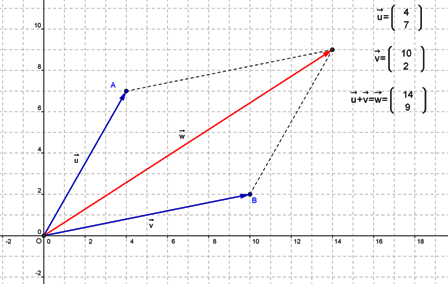The addition of two vectors is represented by the diagonal of the parallelogram constructed using the vectors as the sides. When the vectors are two-dimensional, adding them is analogous to adding two complex numbers.

Vector addition is useful in modelling some combinations of two forces, journeys (displacements) or other vectors.

Vector addition is associative and commutative.